# C博客作业05-指针

| 这个作业属于哪个班级 |

| ---- | ---- | ---- |
| 这个作业的地址 |
| 这个作业的地址这个作业的目标 | 学习指针相关内容 | |
| 姓名 | 王鑫 | |

# 1.本章学习总结

## 1.1指针定义、只针对相关运算、指针做函数参数。

### 1.1.1指针定义

类型名 *指针变量名；
int *p;//定义一个指针变量p，指向整型变量
char *q;//定义一个指针变量q，指向字符型变量
double *a;//定义一个指针变量a，指向双精度实型变量
/*类型名指定指针变量所指向的变量的类型，必须为有效的数据类型。

/*指针变量必须要有指向才可以使用，不然就会成为野指针。

int i,*p;
p=&i;
p=0;//指针可以赋值0，叫做空指针，不指向任何单元
p=NULL;//这个也是空指针
p=(int *)1732;//前面（int*）为强转类型为int的地址，1723。
//可以两个指针指向同一个变量
int a,*p,*q;
p=q=&a;


### 1.1.2指针的基本运算

• 1.取地址运算和间接访问运算
'&'为取地址符，像它的名称一样，'&'的使用就是把变量的地址算出来
int *p,a=3;
p=&a;

'*'就可以访问指针所指向的变量，是间接访问运算符
*p=a;//这时*p和a访问用一个存储单元


### 1.1.3指针做函数参数

• 指针可以作为函数参数传进函数里，而且传入的代价小，并且在函数运算时，可以改变指针指向的变量。
• 可以打破函数一次只能返回一个值的弱点。//根据指针可以改动指针指向的内容的特点
void fiction(int n)
{
n+=1;//一旦函数结束，n值没有变。这里的n和传进来的n不是同一个，生命周期也是随着函数结束而结束。
}
void fiction(int *n)
{
*n+=1;//使用函数就可以改变这个地址内的n值，n的生命周期是随着主函数一起的。这样就不会随着函数而消失。
}


## 1.2字符指针

• 字符串可以看成一个一维的字符数组，而它的数组名就是这个字符串的首地址。
• 我们存放多个字符串的时候就可以设置指针数组来存放多个字符串的首地址。
char sa[]="array ";
char *sp="point ";
printf("%s",sa);//%s把以sa为首地址到遇到'\0'为止的内容输出
printf("%s",sp);
printf("%s\n","string");

array point string

printf("%s",sa+2);//以sa的地址作为首地址输出


### 1.3指针做函数返回值

这里设置找我们想要的字符，找到返回地址
char *find(char *str,char key)
{
for(;*str;str++)
{
if(key==*str)
return str;//因为函数类型设置为指针，我们返回的时候就要返回一个地址回去
}
}

• 有时候我们需要保存我们输进来的值，我们就另设一个指针变量来存放这个进来的地址，保证不会被我们的运算，找不回原来的地址。

## 1.4动态内存分配

### 1.4.2堆区和栈区的区别

• 栈区（stack）函数运行时分配，函数结束时释放。由编译器自动分配释放，存放为运行函数而分配的局部变量、函数参数、返回地址等。
• 堆区（heap）一般由程序员分配释放，若程序员不释放，可能导致内存泄漏，内存碎片化。
• 栈的空间小，如果数据过大超过栈空间，程序会报错栈溢出。堆由程序员自己申请大小，最大不超过主机剩余空间。栈由系统分配，函数结束时释放。

#include<stdlib.h>//要申请动态空间要配上这个头函数
int *p=(int*)malloc(4);
int *p=(int*)malloc(sizeof(int));



### 1.4.3多个字符串做动态内存

char *str[n];
str=(char*)malloc(size(char)*max*n)//我们申请字符串最大可能出现的最大情况
fgets(数组名，长度，stdin）;//今天我用fgets输入，没被执行就跳过了，上网查询过，发现如果前面输入时有'\n'就有可能被下面的fgets吸收，需要注意。


## 1.5指针数组及其应用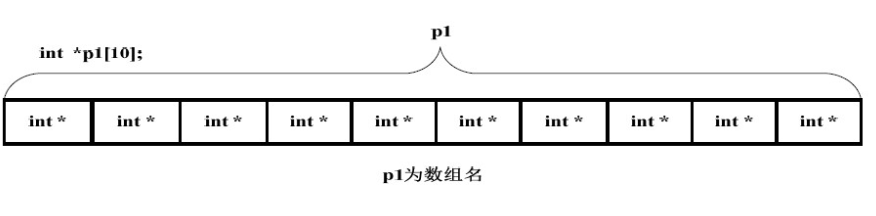• 定义
char *p;//有十个字符型的指针组成的数组
int *a;//有十个整型的指针组成的数组

• 指针数组的空间分配
char *p;//这个p数组本身分配在栈上，对里面的数组成员进行空间分配
p=(char *)malloc（sizeof(char)*max*5);//动态申请空间,这样申请的空间是连续的
for(int i=0;i<m;i++)
a[i]=(char *)malloc(sizeof(char) *n);//这样申请的空间不连续。

• 多个字符串用二维数组表示和用指针数组表示区别
二维数组的长度是固定的，指针数组我们可以调整它每一行的长度。

char* p;
char a;
p = (char *)malloc(sizeof(char) * 10);
strcpy(p, "hello ");
strcpy(a, "world");
printf("%s", p);
printf("%s", a);

p = (char*)malloc(sizeof(char) * 10);
strcpy(p, "1234 ");
strcpy(a, "5678");
printf("%s", p);
printf("%s", a+1);

p = (char*)malloc(sizeof(char) * 10);
strcpy(p, "1234 ");
strcpy(a, "5678");

printf(" ****%c****", *(a+1));
printf("*##*%c*###", *(p+1));


## 1.6二级指针

        char** p;
p = (char**)malloc(sizeof(char*) * 10);
p = (char*)malloc(sizeof(char) * 10);
strcpy(p, "hello");
printf("%s", p);
//指针数组的数组名p也就是一个二级指针
//所以这个时候输出只能用  p  不能用   p  ，p是一个二级指针，不能当成一级指针用


## 1.7行指针、列指针

### 1.7.1定义格式

int a={1,3,5,7,9,11,13,15,17,19,21,23};

int (*p)= &a; // 行指针定义法或者int (*p)= a;//行指针定义法
int *p= a;   // 列指针的定义法


### 1.7.2主要用法

//表示二维数组的全部数字，列指针
int a={1,3,5,7,9,11,13,15,17,19,21,23};

int *p= a;

for(; p < a + 12; p++)

{

printf("%d ",*p);

}
//行指针表示二维数组的全部数字
for(i = 0; i < 3; i++)

for(j = 0; j < 4; j++)

{

printf("%d ",*(*(p + i) + j));

}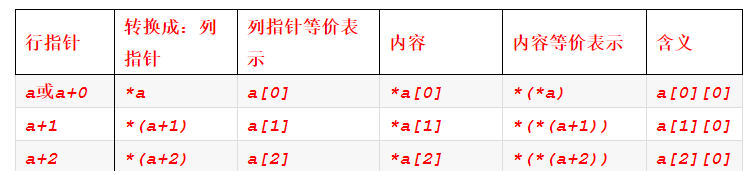# 2.PTA实验作业

## 2.1删除字符串中的子串

### 2.1.1伪代码

先输入s1,s2

for (int i to len1)
if(s1[i]==s2&&len1剩余的长度>len2）

if s1[j]!=s2[k]//有不一样的
flag=0//设立一个标志来看是否要重新检索,这时没有删除字符串，不检索
break；
end if
end for
//在第一个已经判断过的情况下，判断这时有没有删除了字符
if（flag==1）//flag=1代表中途有相同的字符串。

len1-=len2；
i->-1;//代表会循环时重头开始
end



### 2.1.2代码截图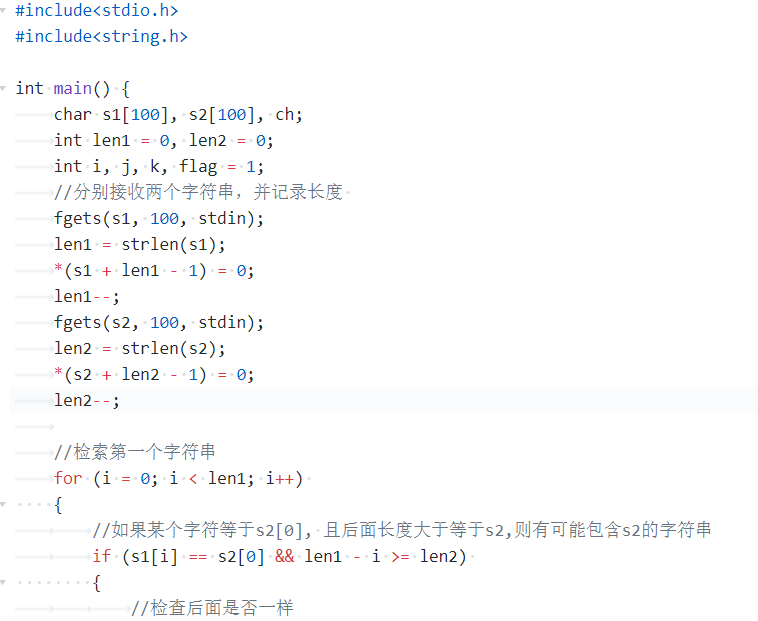### 2.1.3代码比较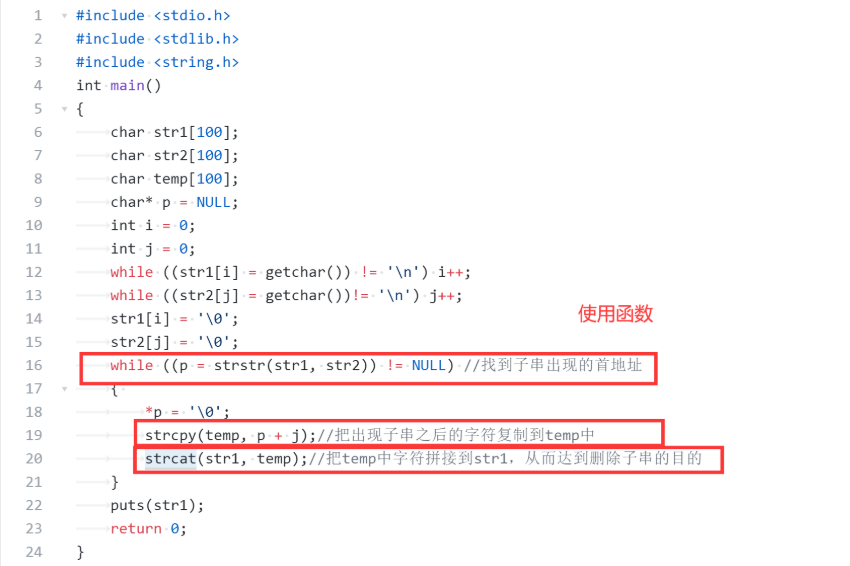同学的代码简洁直观，熟练运用比较多的头文件里的函数，这样让代码量很少，看着也很轻松。



## 2.2合并2个有序数组

### 2.2.1伪代码

if 第一个数组长度为0

else

b往下移一位 j++

end


### 2.2.2代码截图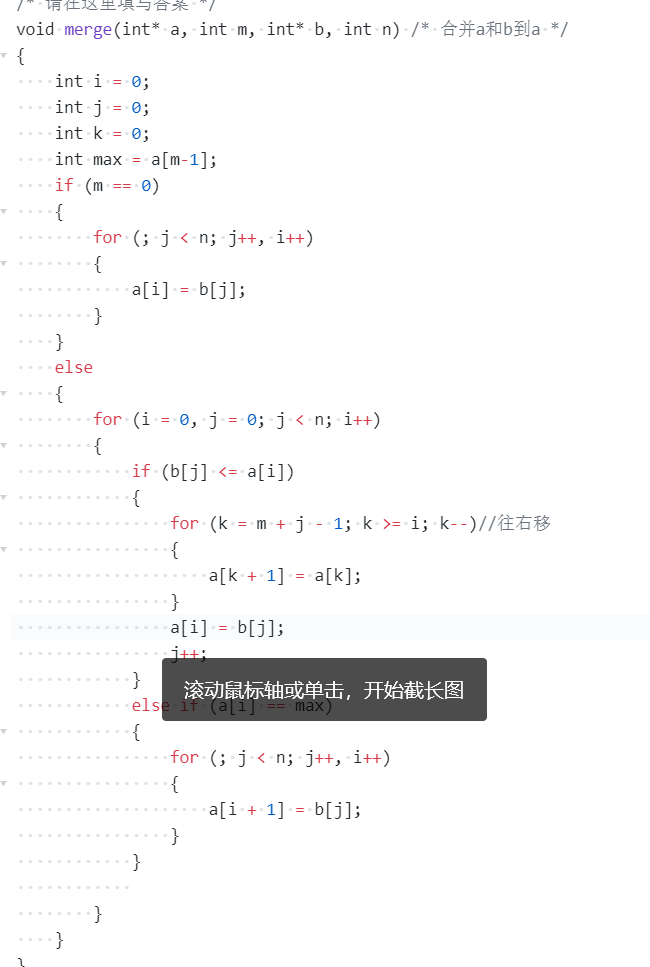### 2.2.3代码对比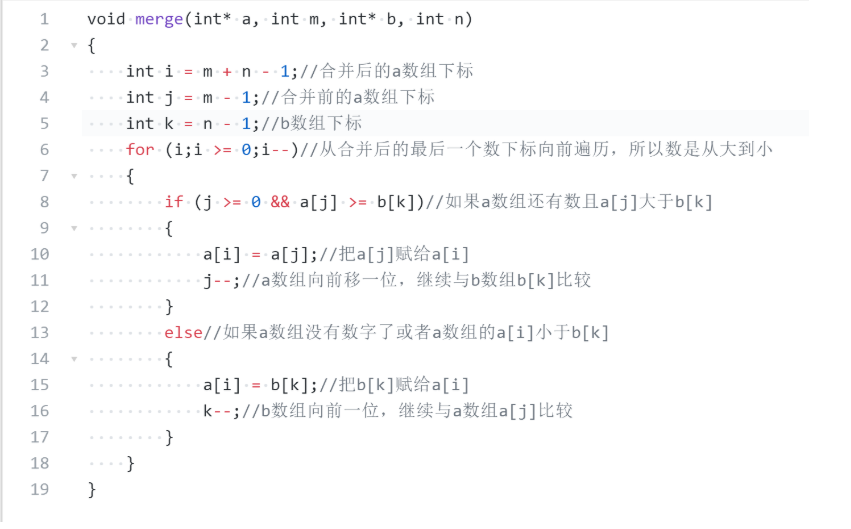同学重构数组，把两个数组之间进行比较，大的放在后面，这个做法省去了像我把数组的数字往后放的时间，而且思路清晰。



## 2.3说反话-加强版

### 2.3.1伪代码

从尾部开始
*p=str+len
for p to str
if(*p=' ')遇见空格看成一个单词

else
count++;



### 2.3.2代码截图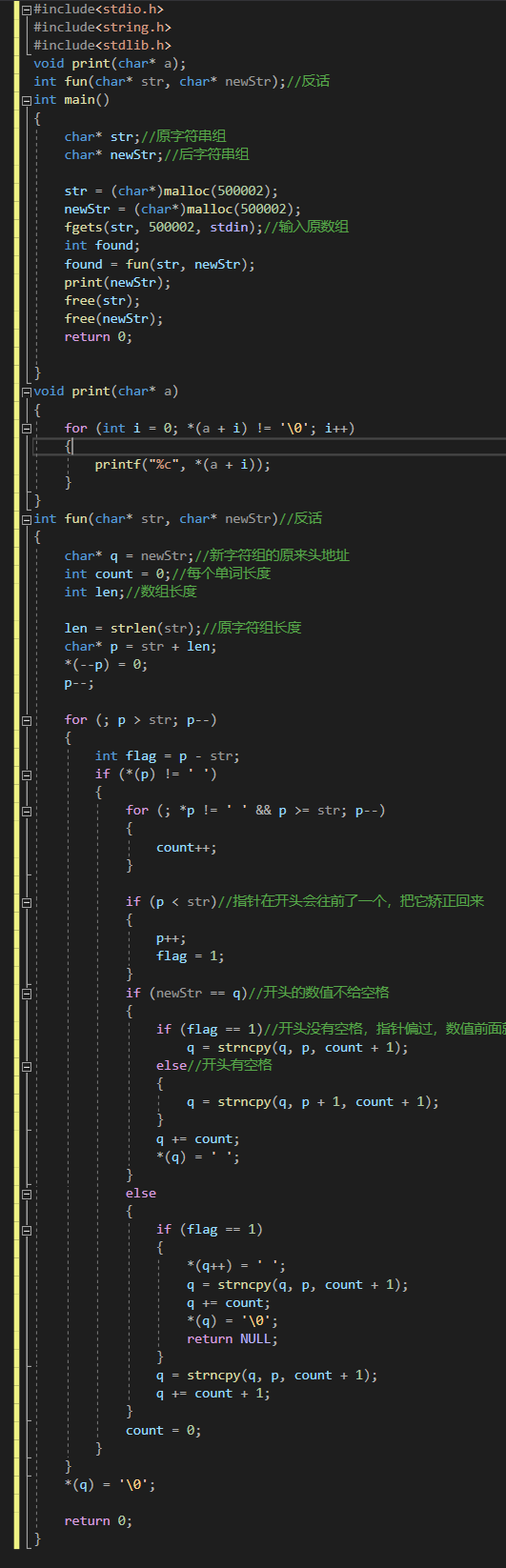### 2.3.3代码比较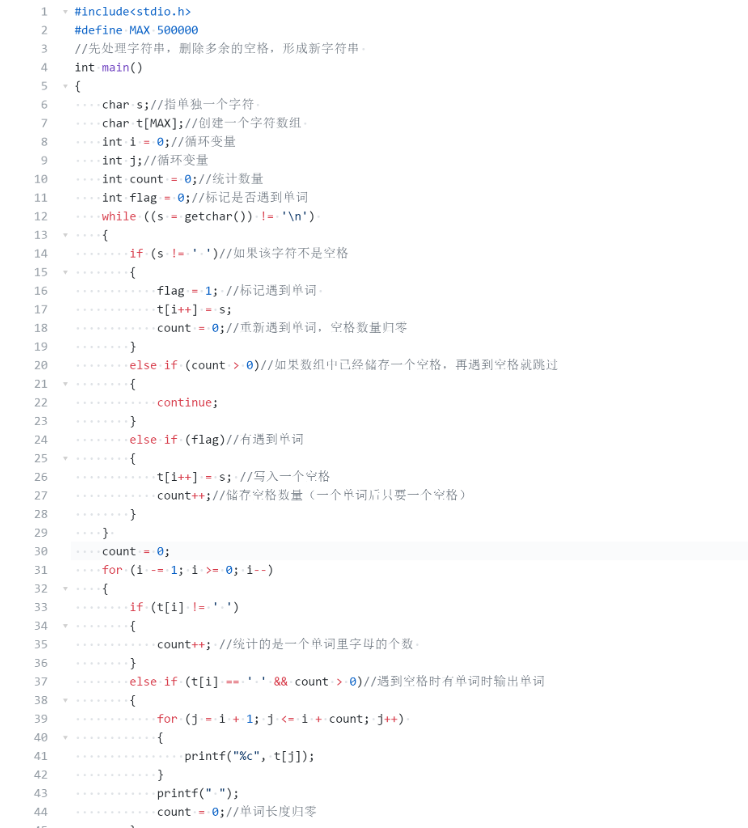同学单独设置了空格的控制，这样更好处理数据，我把空格和单词一起输进新的数组，这样要处理的情况会要多设置几个。



posted @ 2020-12-27 22:53  兴亡  阅读(142)  评论(0编辑  收藏  举报